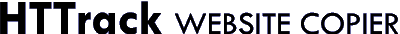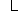Subject: Errors found by valgrind
Author: Lars Clausen
Date: 02/12/2004 17:38
 ```Having had my share of memory bugs, I'm running HTTrack under valgrind now. Apart from having found another bug in my ARC writer, here's some output from running without any plugins. It appears to be just two bugs, one in hts_acceptlink and one in vxgethostbyname, or thereabouts. -Lars ==5971== ==5971== Conditional jump or move depends on uninitialised value(s) ==5971== at 0xB74CB573: strchr (mac_replace_strmem.c:113) ==5971== by 0xB73ACA5D: hts_acceptlink (htswizard.c:198) ==5971== by 0xB732EAC5: htsparse (htsparse.c:2190) ==5971== by 0xB7322149: httpmirror (htscore.c:1448) ==5971== by 0xB73856CF: hts_main (htscoremain.c:2296) ==5971== by 0x8048E18: main (httrack.c:205) ==5971== ==5971== Conditional jump or move depends on uninitialised value(s) ==5971== at 0xB74CB578: strchr (mac_replace_strmem.c:114) ==5971== by 0xB73ACA5D: hts_acceptlink (htswizard.c:198) ==5971== by 0xB732EAC5: htsparse (htsparse.c:2190) ==5971== by 0xB7322149: httpmirror (htscore.c:1448) ==5971== by 0xB73856CF: hts_main (htscoremain.c:2296) ==5971== by 0x8048E18: main (httrack.c:205) ==5971== ==5971== Conditional jump or move depends on uninitialised value(s) ==5971== at 0xB74CB573: strchr (mac_replace_strmem.c:113) ==5971== by 0xB73ACA5D: hts_acceptlink (htswizard.c:198) ==5971== by 0xB739CF19: url_savename (htsname.c:461) ==5971== by 0xB732EC89: htsparse (htsparse.c:2235) ==5971== by 0xB7322149: httpmirror (htscore.c:1448) ==5971== by 0xB73856CF: hts_main (htscoremain.c:2296) ==5971== by 0x8048E18: main (httrack.c:205) ==5971== ==5971== Conditional jump or move depends on uninitialised value(s) ==5971== at 0xB74CB578: strchr (mac_replace_strmem.c:114) ==5971== by 0xB73ACA5D: hts_acceptlink (htswizard.c:198) ==5971== by 0xB739CF19: url_savename (htsname.c:461) ==5971== by 0xB732EC89: htsparse (htsparse.c:2235) ==5971== by 0xB7322149: httpmirror (htscore.c:1448) ==5971== by 0xB73856CF: hts_main (htscoremain.c:2296) ==5971== by 0x8048E18: main (httrack.c:205) ==5971== ==5971== Conditional jump or move depends on uninitialised value(s) ==5971== at 0xADFDE3F4: getanswer_r (in /lib/libnss_dns-2.3.2.so) ==5971== by 0xADFDD0D1: _nss_dns_gethostbyname2_r (in /lib/libnss_dns-2.3.2.so) ==5971== by 0xB725513E: gaih_inet (in /lib/i686/libc-2.3.2.so) ==5971== by 0xB7256463: __GI_getaddrinfo (in /lib/i686/libc-2.3.2.so) ==5971== by 0xB7381DE9: vxgethostbyname (htslib.c:4331) ==5971== by 0xB7382484: hts_gethostbyname (htslib.c:4406) ==5971== by 0xB7378FAE: newhttp (htslib.c:2039) ==5971== by 0xB736C07F: http_xfopen (htslib.c:657) ==5971== ==5971== Conditional jump or move depends on uninitialised value(s) ==5971== at 0xADFDE3FA: getanswer_r (in /lib/libnss_dns-2.3.2.so) ==5971== by 0xADFDD0D1: _nss_dns_gethostbyname2_r (in /lib/libnss_dns-2.3.2.so) ==5971== by 0xB725513E: gaih_inet (in /lib/i686/libc-2.3.2.so) ==5971== by 0xB7256463: __GI_getaddrinfo (in /lib/i686/libc-2.3.2.so) ==5971== by 0xB7381DE9: vxgethostbyname (htslib.c:4331) ==5971== by 0xB7382484: hts_gethostbyname (htslib.c:4406) ==5971== by 0xB7378FAE: newhttp (htslib.c:2039) ==5971== by 0xB736C07F: http_xfopen (htslib.c:657) ==5971== ==5971== Conditional jump or move depends on uninitialised value(s) ==5971== at 0xADFDE3F4: getanswer_r (in /lib/libnss_dns-2.3.2.so) ==5971== by 0xADFDD0D1: _nss_dns_gethostbyname2_r (in /lib/libnss_dns-2.3.2.so) ==5971== by 0xB7255254: gaih_inet (in /lib/i686/libc-2.3.2.so) ==5971== by 0xB7256463: __GI_getaddrinfo (in /lib/i686/libc-2.3.2.so) ==5971== by 0xB7381DE9: vxgethostbyname (htslib.c:4331) ==5971== by 0xB7382484: hts_gethostbyname (htslib.c:4406) ==5971== by 0xB7378FAE: newhttp (htslib.c:2039) ==5971== by 0xB736C07F: http_xfopen (htslib.c:657) ==5971== ==5971== Conditional jump or move depends on uninitialised value(s) ==5971== at 0xADFDE3FA: getanswer_r (in /lib/libnss_dns-2.3.2.so) ==5971== by 0xADFDD0D1: _nss_dns_gethostbyname2_r (in /lib/libnss_dns-2.3.2.so) ==5971== by 0xB7255254: gaih_inet (in /lib/i686/libc-2.3.2.so) ==5971== by 0xB7256463: __GI_getaddrinfo (in /lib/i686/libc-2.3.2.so) ==5971== by 0xB7381DE9: vxgethostbyname (htslib.c:4331) ==5971== by 0xB7382484: hts_gethostbyname (htslib.c:4406) ==5971== by 0xB7378FAE: newhttp (htslib.c:2039) ==5971== by 0xB736C07F: http_xfopen (htslib.c:657) ```

All articles

 Subject Author Date Errors found by valgrind Lars Clausen 02/12/2004 17:38Re: Errors found by valgrindXavier Roche 02/14/2004 12:26

3

Created with FORUM 2.0.11

 © 2020 Xavier Roche & other contributors - Web Design: Leto Kauler.